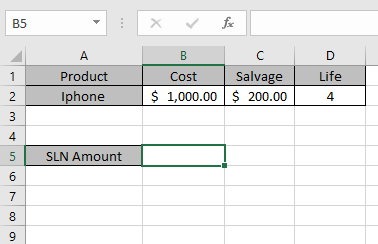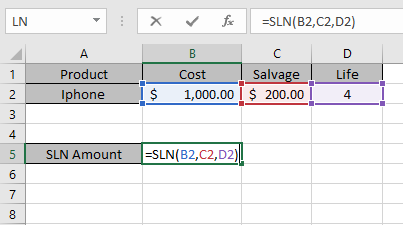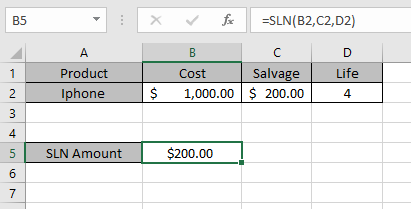# How to use the SLN Function in Excel

In this article, we will learn about how to use the SLN function in Excel.

SLN function is Straight line depreciation method which is the most simple way of calculating the depreciation of any asset. The process simply subtracts a fixed amount from the value of an asset during each period of the asset's lifetime. Therefore, the straight line depreciation for one period is calculated by the following equation:

SLN = (cost - salvage) / life

The SLN function returns the depreciation amount for the input data
Syntax:

=SLN (cost, salvage, life)

Cost : Cost price of the asset
Salvage : remaining price of the asset after a period
Life : period after which cost price becomes salvage price

Let’s understand this function using it in an example.
Here we took a product as Iphone. The cost price & salvage price of the latest Iphone is shown in the snapshot below.Now we need to calculate the depreciation amount for the Iphone of every year.

Use the formula:

=SLN(B2,C2,D2)

B2 : Cost price of the asset
C2 : remaining price of the asset after a period
D2 : 4 years after cost price becomes salvage priceThe values to the formula are taken as cell reference.As you can see from the above returned value, every year \$ 200 will depreciated from the asset cost value.

 Year Depreciation Salvage value 0 0 \$ 1000 1 \$ 200 \$ 800 2 \$ 200 \$ 600 3 \$ 200 \$ 400 4 \$ 200 \$ 200

You can see from the above table that depreciation amount is deducted from the salvage amount for every year up till life of an asset

Note:

1. The function generates #VALUE! Error if arguments to the function is non - numeric
2. The function generates #NUM! Error if:
• The value of cost and salvage is less than 0.

The value of life of asset is less than or equals to zero.

Hope you understood how to use SLN function and referring cell in Excel. Explore more articles on Excel financial functions here. Please feel free to state your query or feedback for the above article.

Related Articles:

How to use the DB Function in Excel

How to use the DDB Function in Excel

How to use the SYD Function in Excel

Popular Articles:

50 Excel Shortcuts to Increase Your Productivity

How to use the VLOOKUP Function in Excel

How to use the COUNTIF in Excel 2016

How to use the SUMIF Function in Excel

Terms and Conditions of use

The applications/code on this site are distributed as is and without warranties or liability. In no event shall the owner of the copyrights, or the authors of the applications/code be liable for any loss of profit, any problems or any damage resulting from the use or evaluation of the applications/code.